# How to create a mortgage calculator in Microsoft Excel (21 Things to Know)## How to create a mortgage calculator in Microsoft Excel

When it comes to mortgage-related expenses like interest and monthly payments, one of the most useful features of Excel is its ability to calculate them.

Even if you are not highly familiar with Excel operations, creating a mortgage calculator in Excel is a straightforward process.

This article will show you how to design a mortgage calculator as well as an amortization schedule in Microsoft Excel using the formulas provided.

Method No. 1

### #1. Creating a Mortgage CalculatorOpen the spreadsheet program on Microsoft Excel. If you don’t have Excel installed on your computer, you can substitute Outlook’s online Excel extension for the functionality of the spreadsheet program. It’s possible that you’ll need to create an Outlook account first.Choose a blank workbook from the drop-down menu. This will create a new Excel spreadsheet on your browser.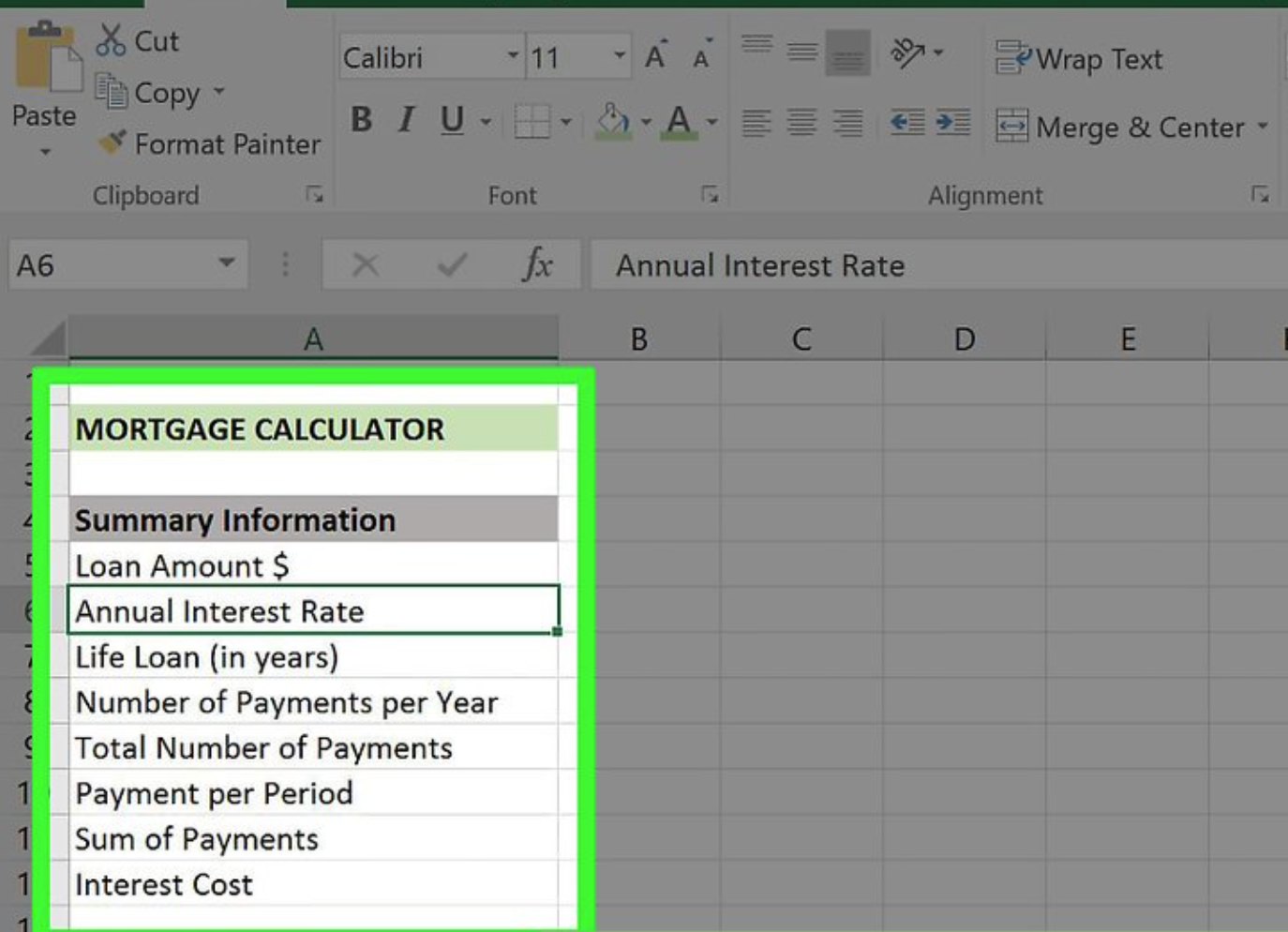Create a column for “Categories”  This will go in the “A” column of the spreadsheet.
This will require first moving the separator between columns “A” and “B” to the right at least three spaces in order to ensure that you do not run out of space to write on the next page. There will be a total of eight cells required for the following categories:
• Loan Amount \$
• Annual Interest Rate
• Life Loan (in years)
• Number of Payments per Year
• Total Number of Payments
• Payment per Period
• Sum of Payments
• Interest Cost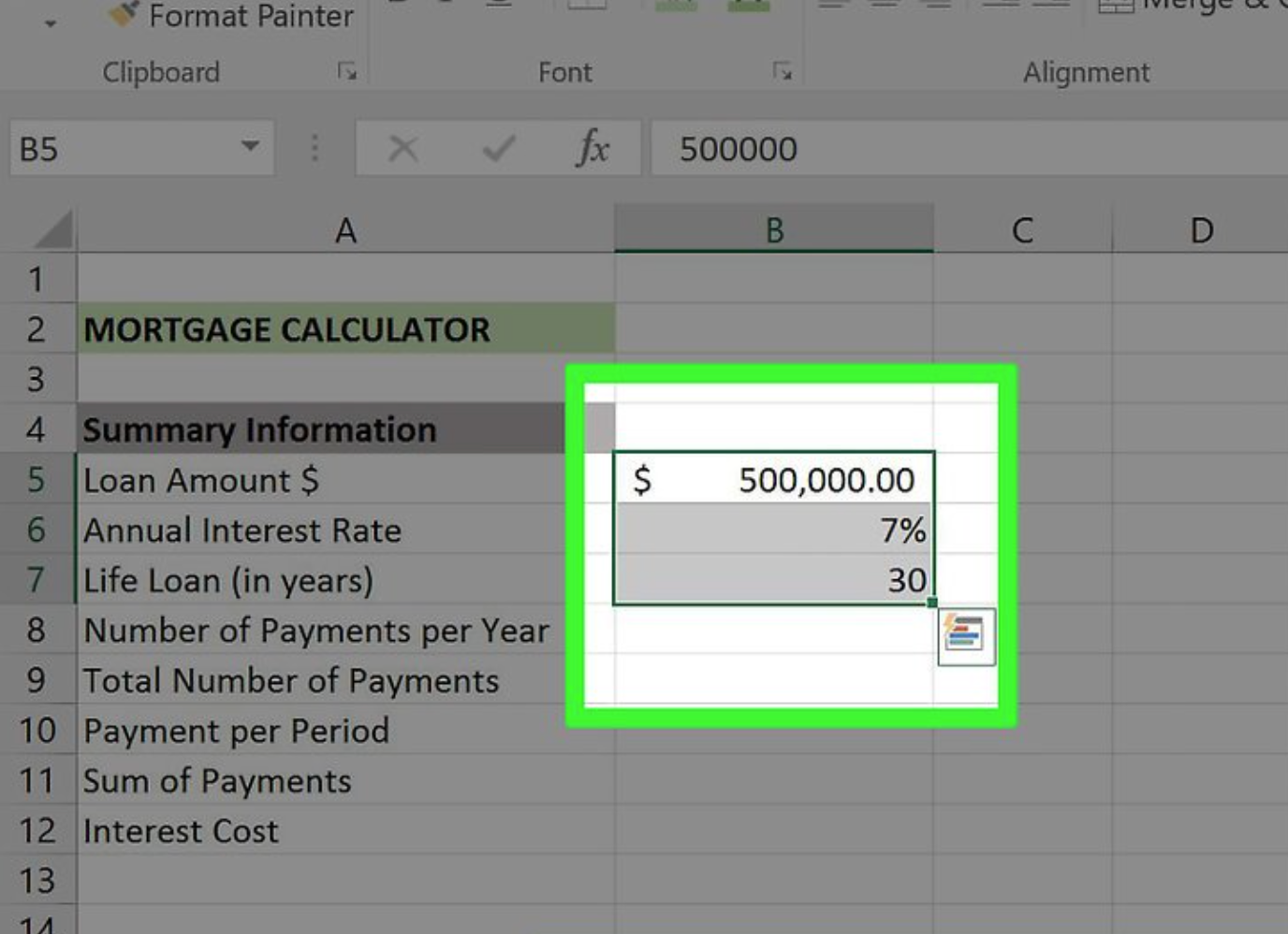Enter your values. All of them will be placed in your “Bcolumn, which will be just to the right of your “Categories” column.

You’ll need to fill in the blanks with the right numbers for your mortgage.

• Your Loan Amount value is the total amount you owe.
• Your Annual Interest Rate value is the percentage of interest that accrues each year.
• Your Life Loan – The loan’s worth is the period of time you have to pay it off, expressed in years.
• Your Number of Payments per Year value is how many times you make a payment in one year.
• Your Total Number of Payments value is the Life Loan value multiplied by the Payments Per Year value.
• Your Payment per Period value is the amount you pay per payment.
• Your Sum of Payments value covers the total cost of the loan.
• Your Interest Cost value determines the total cost of the interest over the course of the Life Loan value.
5 Smart Ways to Drastically Lower Your Electric Bill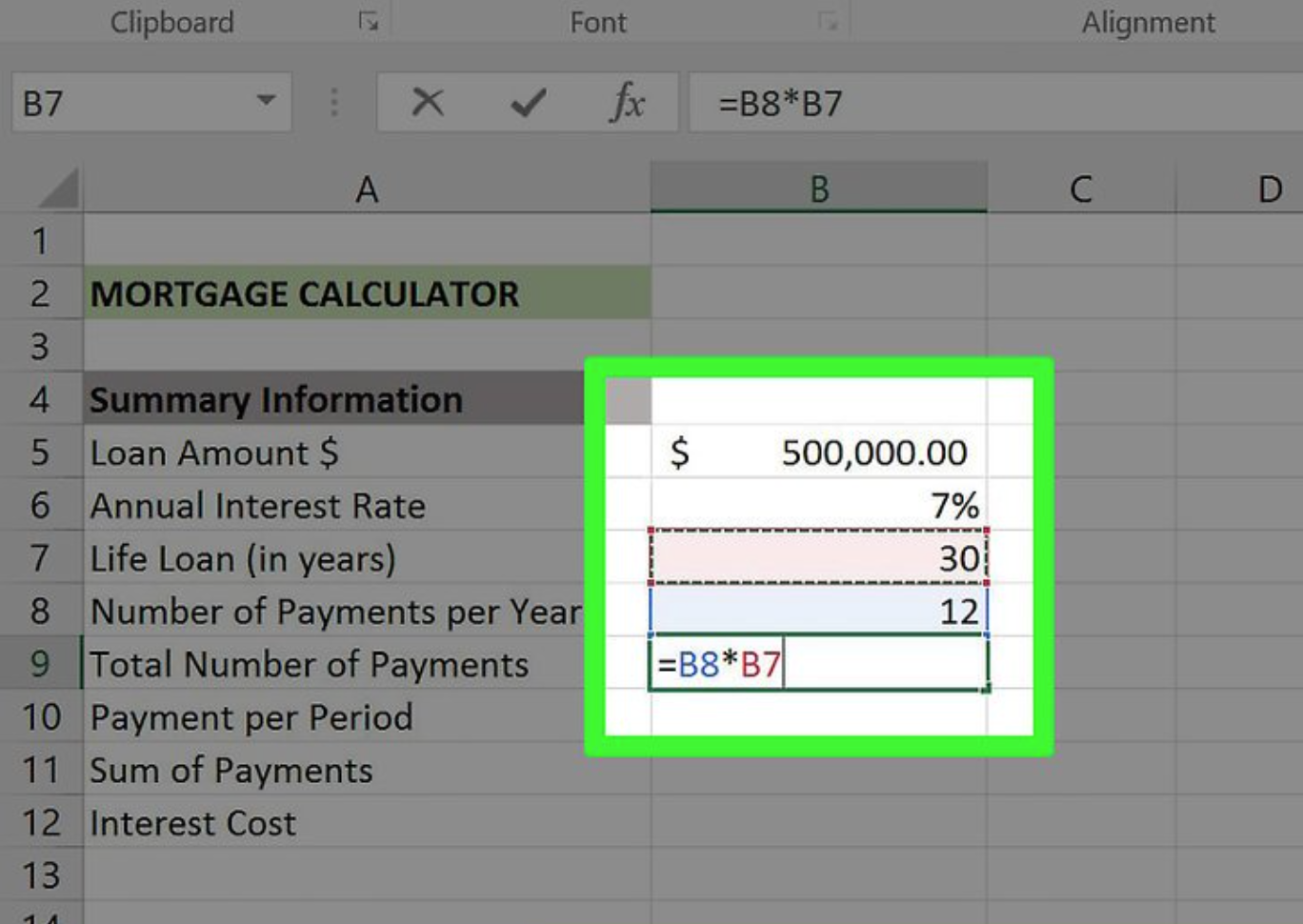Calculate the total number of payments that will be made. It is not necessary to use a formula to compute this amount because it is equal to your Life Loan value multiplied by your Payments Per Year value.

You would enter “360” in this field if you were making a payment every month on a 30-year life loan.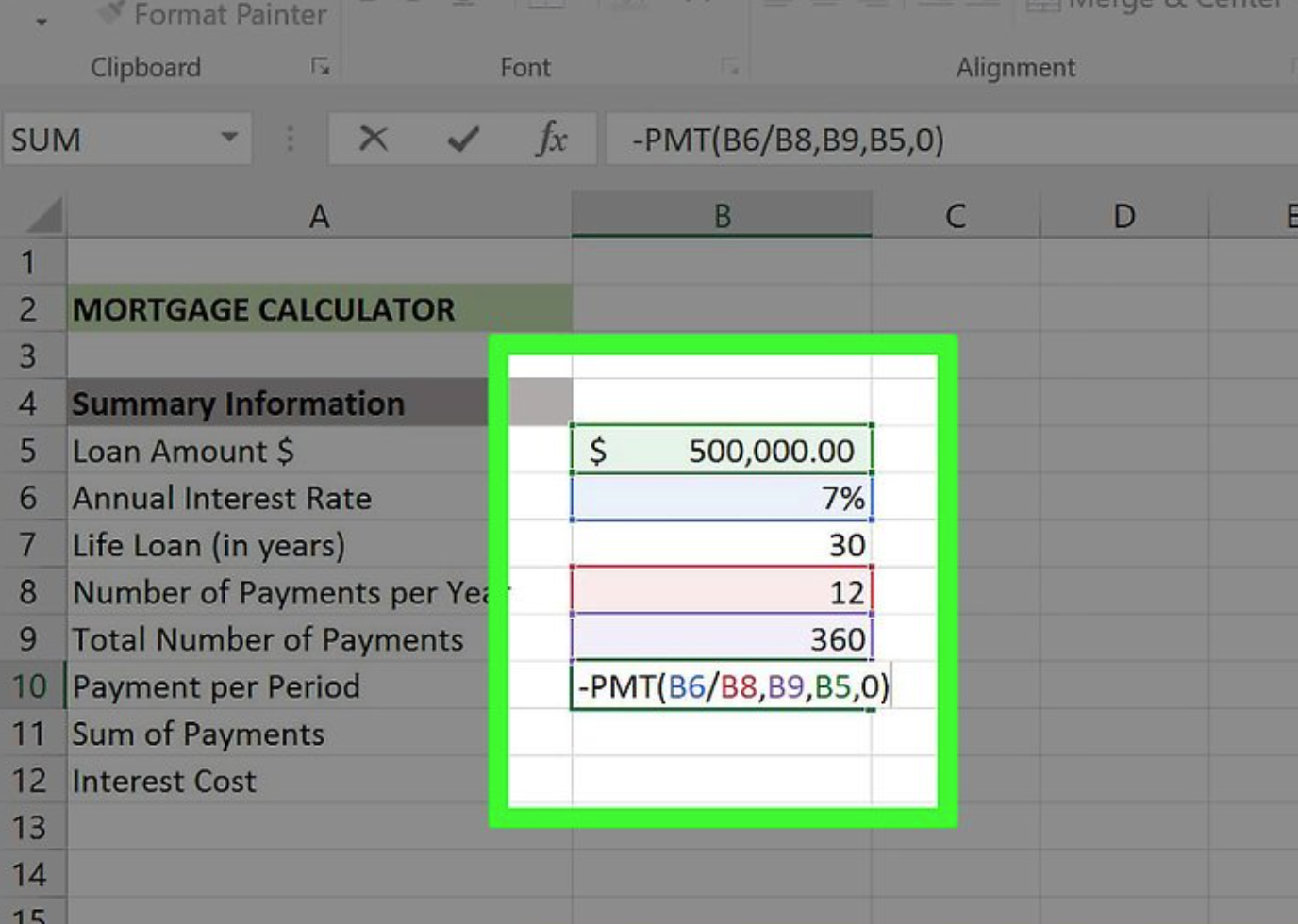The formula is “-PMT(B6/B8,B9,B5,0)” in the screenshot supplied above. If your values are somewhat different from the ones in the table, enter them in the corresponding cell numbers.

When you place a negative sign in front of PMT, this means that PMT refunds the amount that will be taken from the amount owing to the company.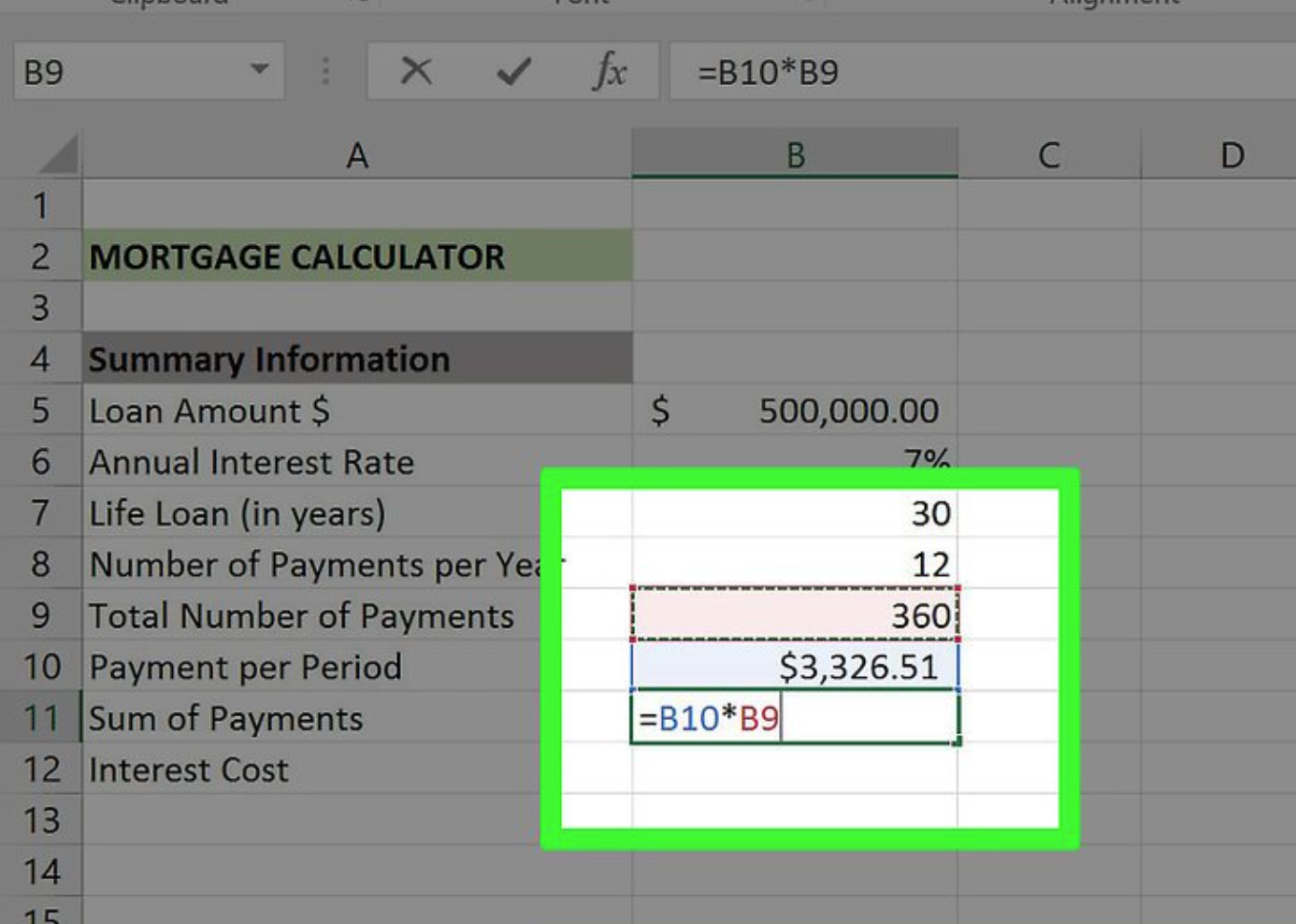Calculate the total cost of the loan.

To do so, simply multiply the value of your “payment per period” by the value of your “total number of payments” value.

Consider the following example: if you make 360 payments of \$600.00 each, the total cost of the loan will be \$216,000.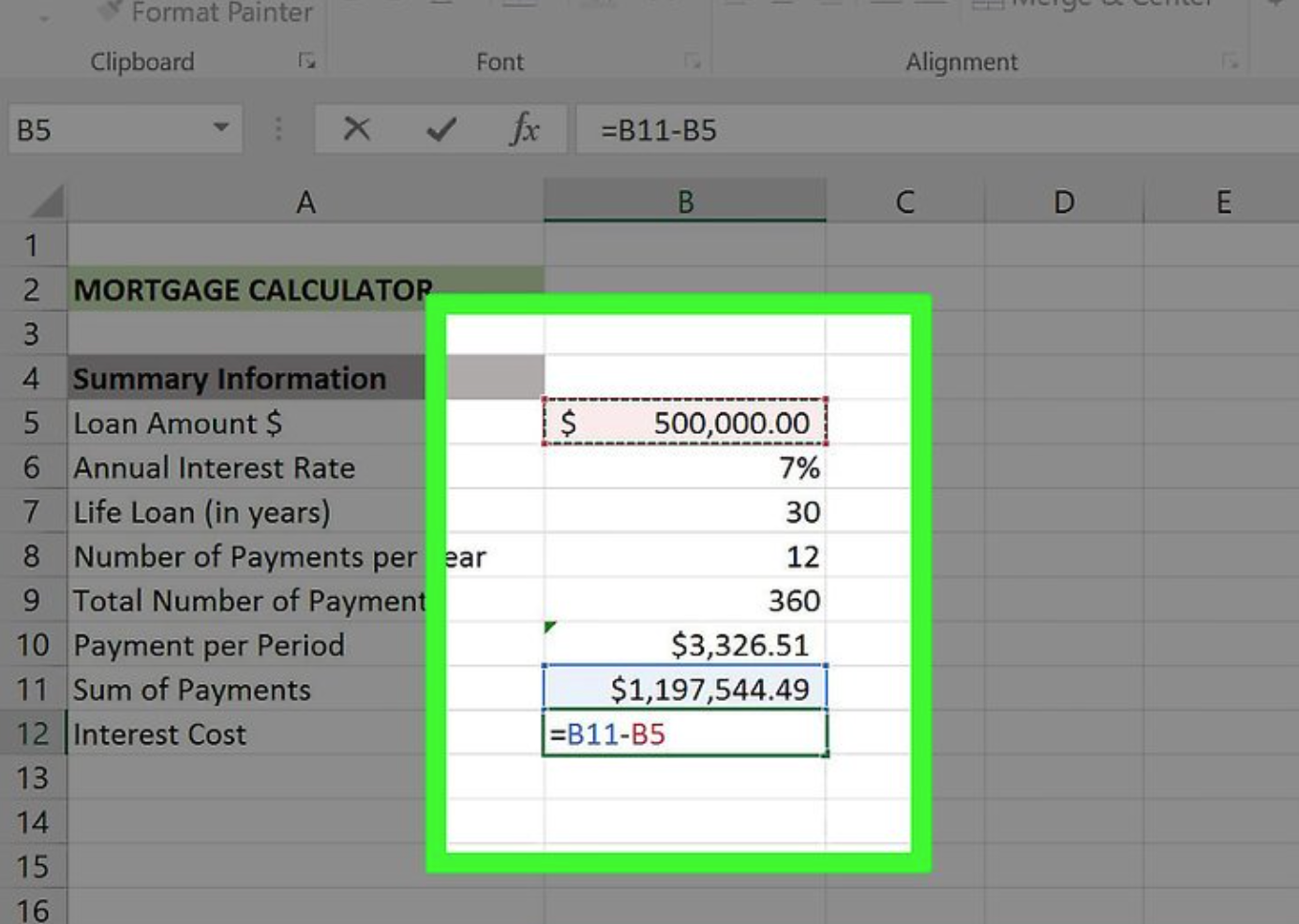Calculate the total interest cost. All you have to do now is subtract the amount of your initial loan from the overall cost of the loan, which you estimated previously. After you’ve completed this step, your mortgage calculator will be complete.

### Making a Payment Schedule (Amortization)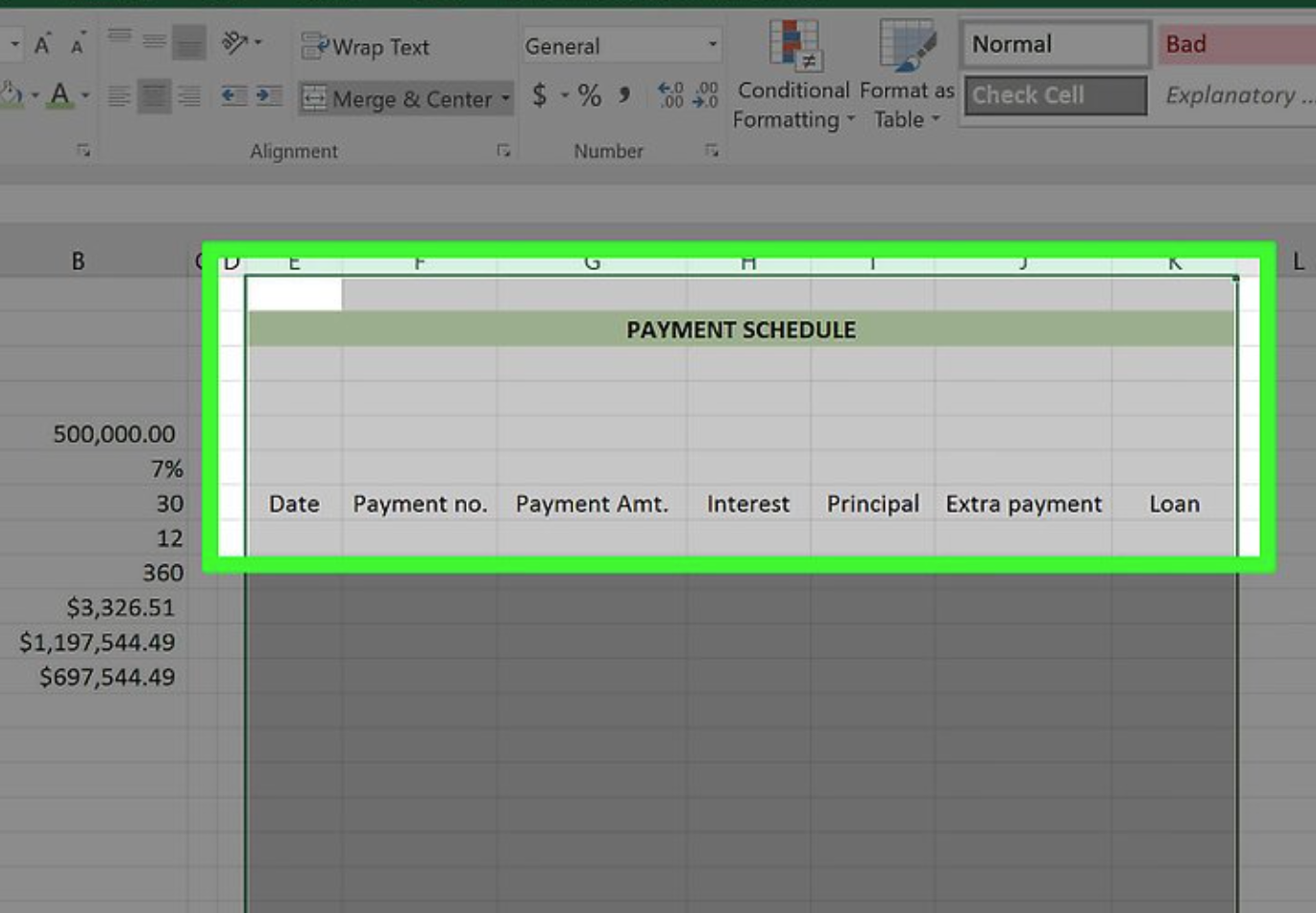Create your Payment Schedule template to the right of your Mortgage Calculator template.

Because the Payment Schedule relies on the Mortgage Calculator to provide you with an accurate estimate of how much you’ll owe/pay off each month, both documents should be included in the same package. It is necessary to have a separate column for each of the following categories:

• Date – The date the payment in question is made.
• Payment (number) – The payment number out of your total number of payments (e.g., “1”, “6”, etc.).
• Payment (\$) – The total amount paid.
• Interest – The amount of the total paid that is interest.
• Principal – The amount of the total paid with no interest (e.g., loan payment).
• Extra Payment – The dollar amount of any extra payments you make.
• Loan – The amount of your loan that remains after a payment.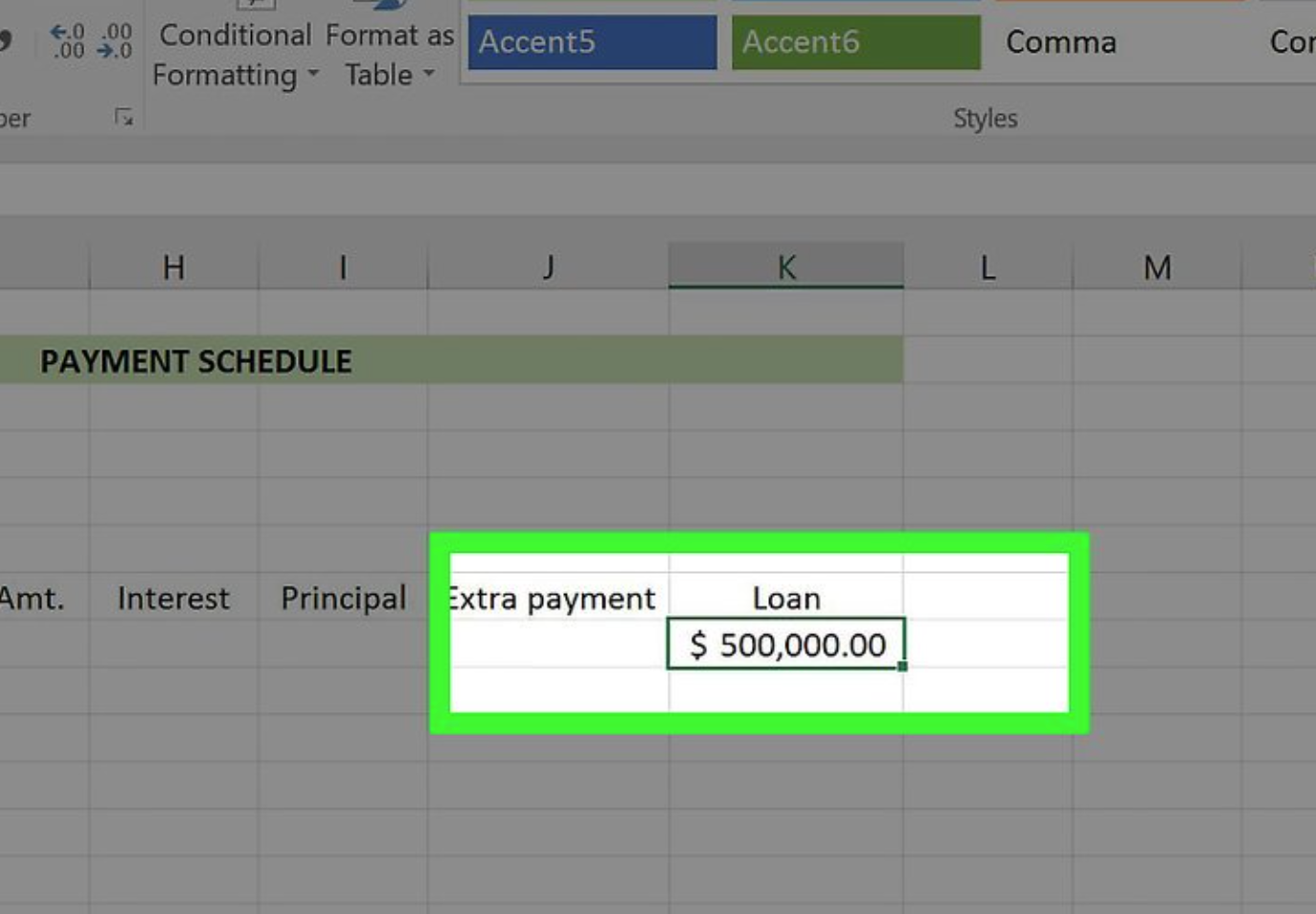Add the original loan amount to the payment schedule. In the “Loan” column, this will be placed in the first vacant cell at the very top.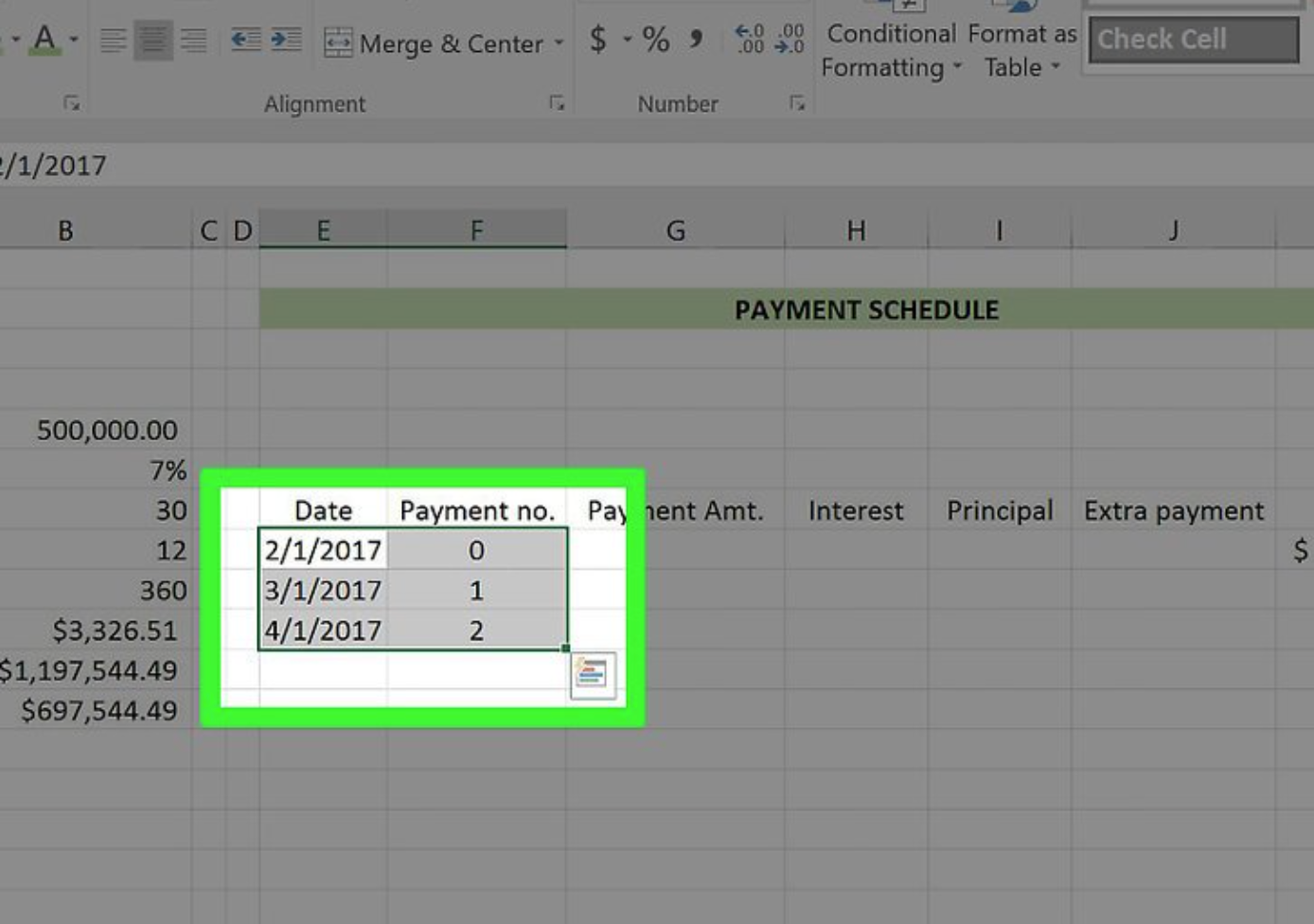Set up the first three cells in your “Date” and “Payment (Number)” columns.

To complete the date column, you’ll need to fill in the date on which you took out the loan, as well as the first two dates on which you intend to make the monthly payment (for example, 2/1/1999, 3/1/1999, and 4/1/1999).
In the Payment column, enter the first three payment numbers that have been received (e.g., 0, 1, 2).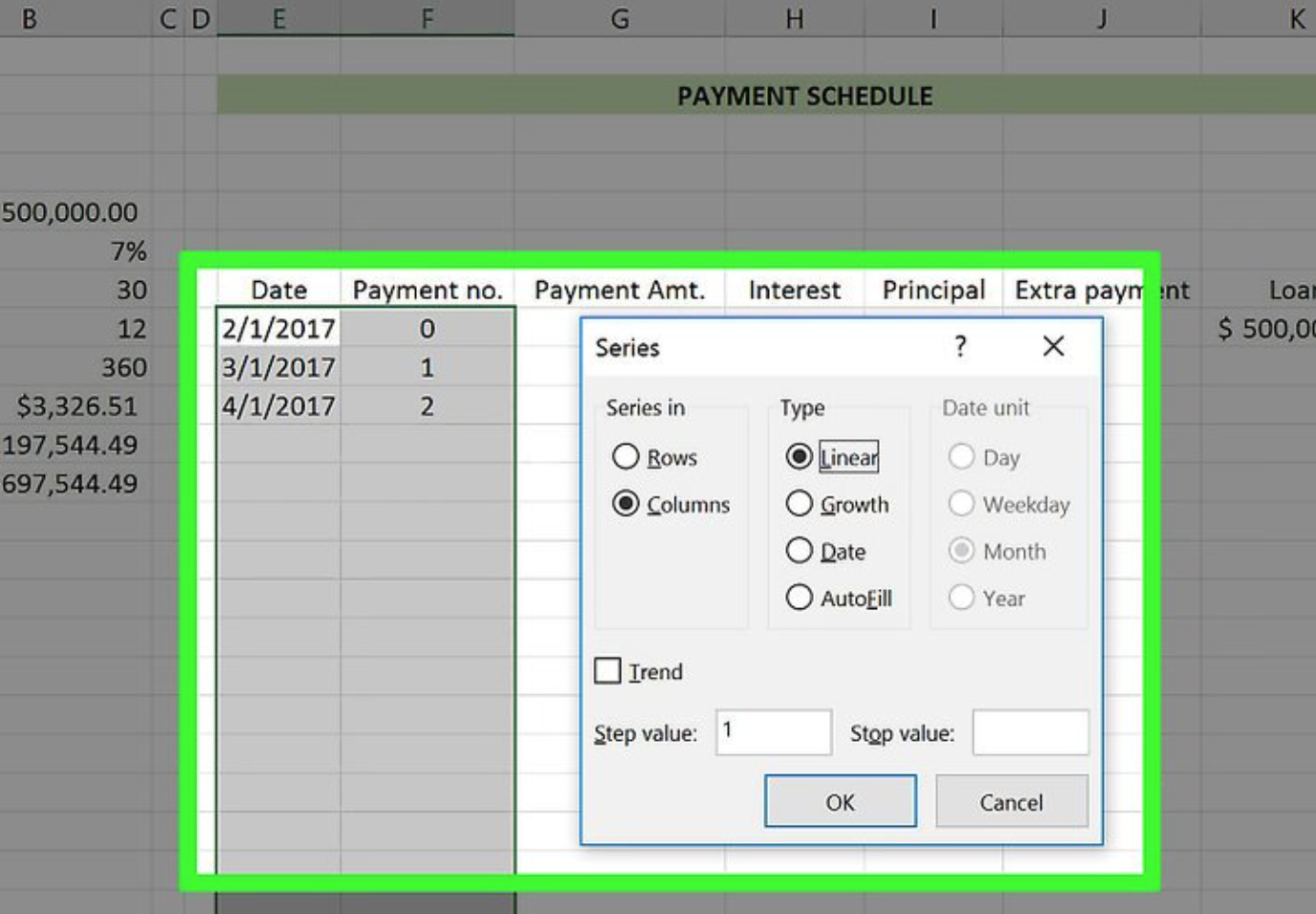Use the “Fill” function to automatically enter the rest of your Payment and Date values. To do so, you’ll need to perform the following steps: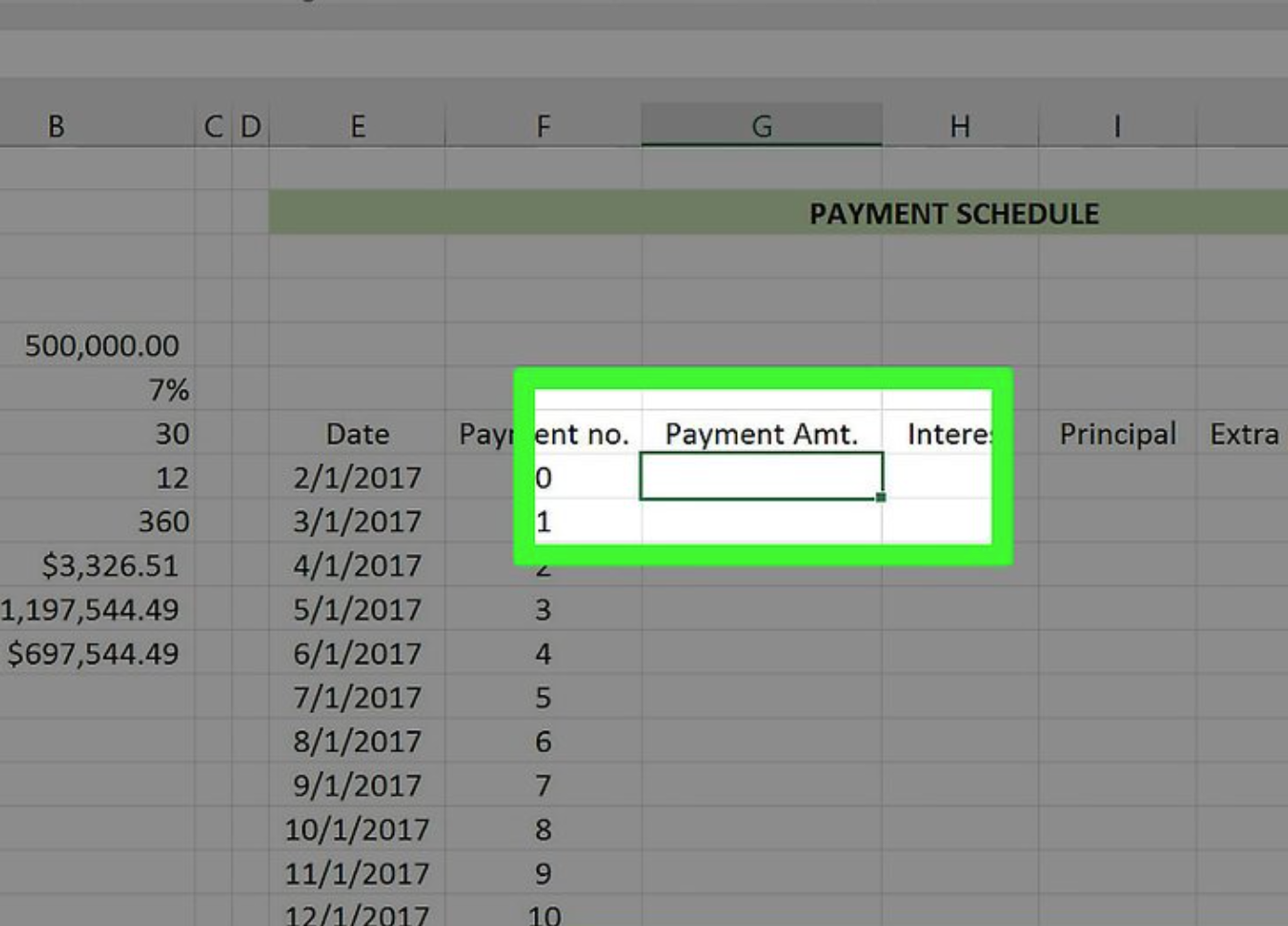Select the first empty cell in the “Payment (\$)” column.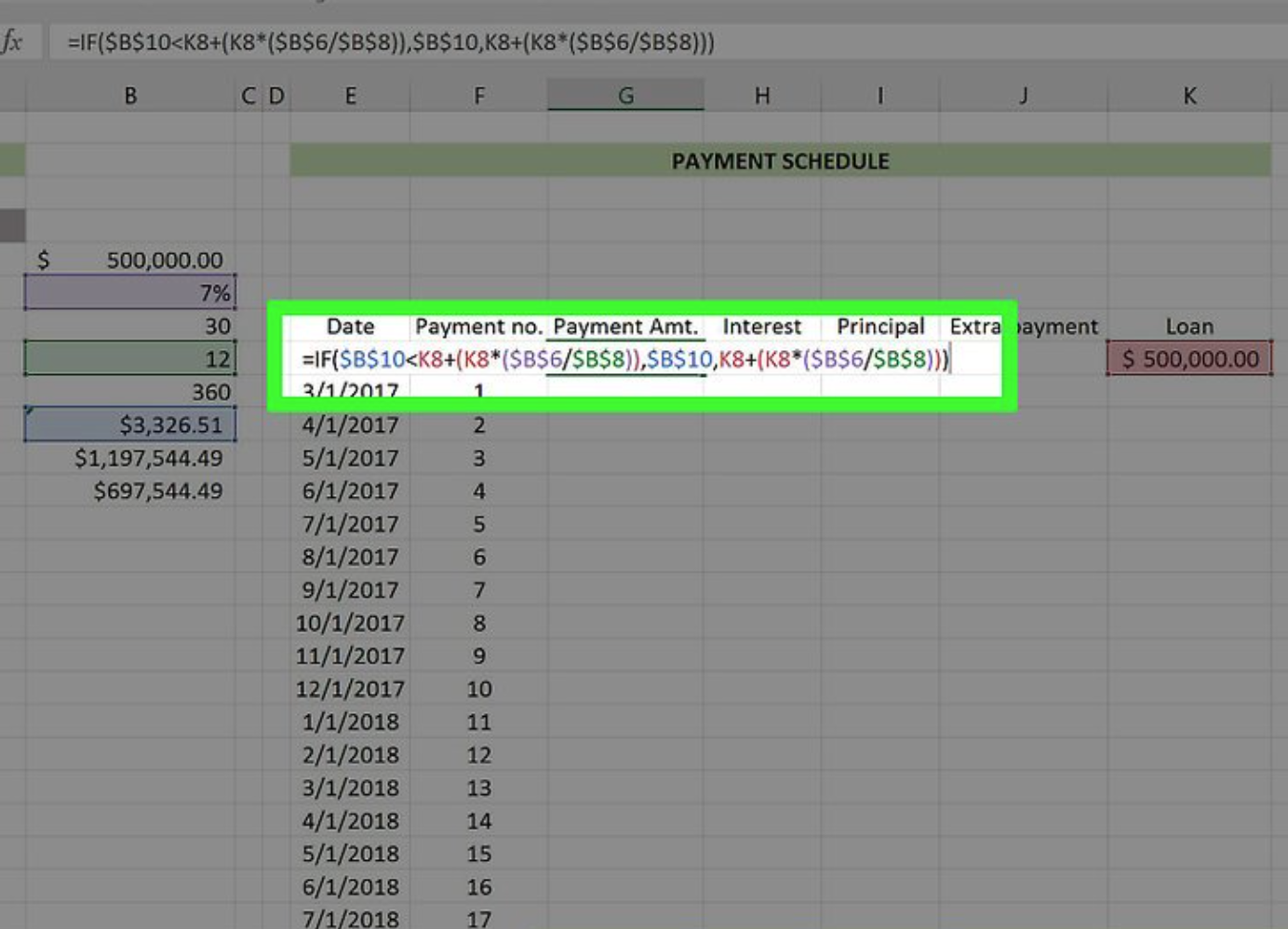Enter the Payment per Period formula. The formula for calculating your Payment per Period value is as follows:
“Payment per Period, Total Loan+(Total Loan*(Annual Interest Rate/Number of Payments per Year)), Payment per Period, Total Loan+(Total Loan*(Annual Interest Rate/Number of Payments per Year)), Payment per Period, Total Loan+(Total Loan*(Annual Interest Rate/Number of Payments per Year))).
• You must preface this formula with the “=IF” tag to complete the calculations.
• Your “Annual Interest Rate”, “Number of Payments per Year”, and “Payment per Period” values will need to be written like so: \$letter\$number. For example: \$B\$6
• Given the screenshots here, the formula would look like this: “=IF(\$B\$10<K8+(K8*(\$B\$6/\$B\$8)),\$B\$10, K8+(K8*(\$B\$6/\$B\$8)))” (sans the quotation marks).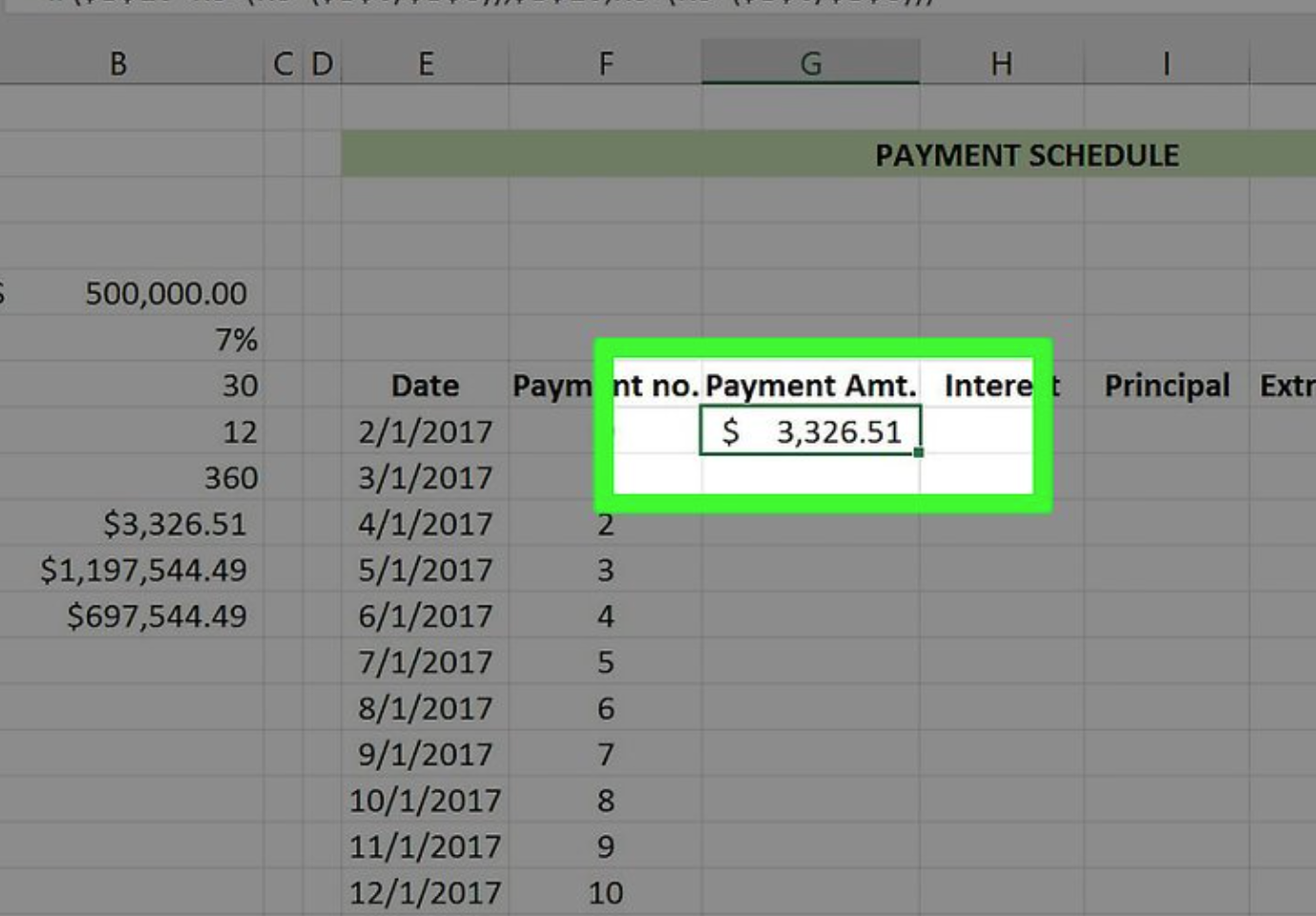Press Enter.

This will populate the specified cell with the Payment per Period formula.

To ensure that this formula is applied to all consecutive cells in this column, you must employ the “Fill” option that you previously used.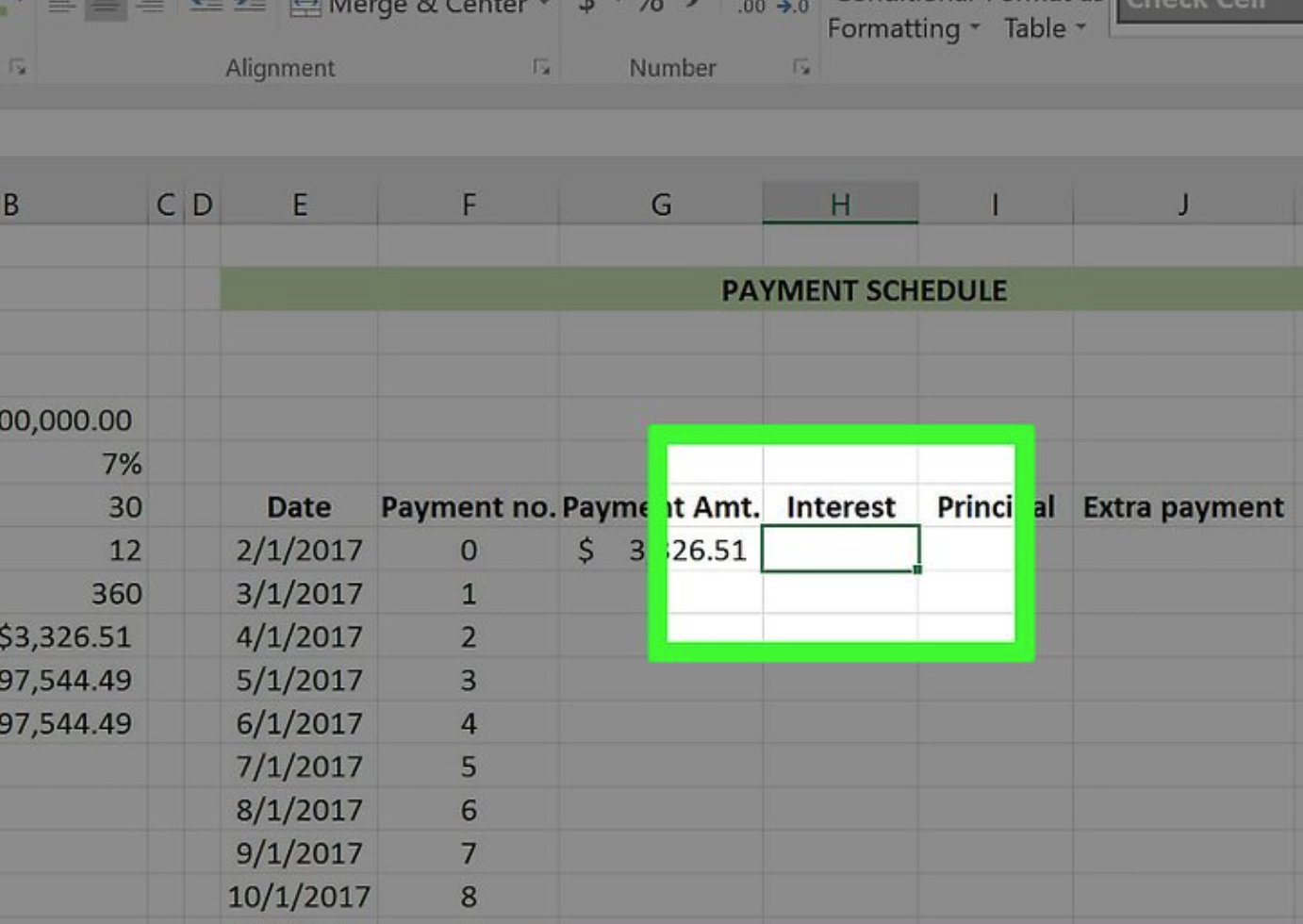Select the first empty cell in the “Interest” column.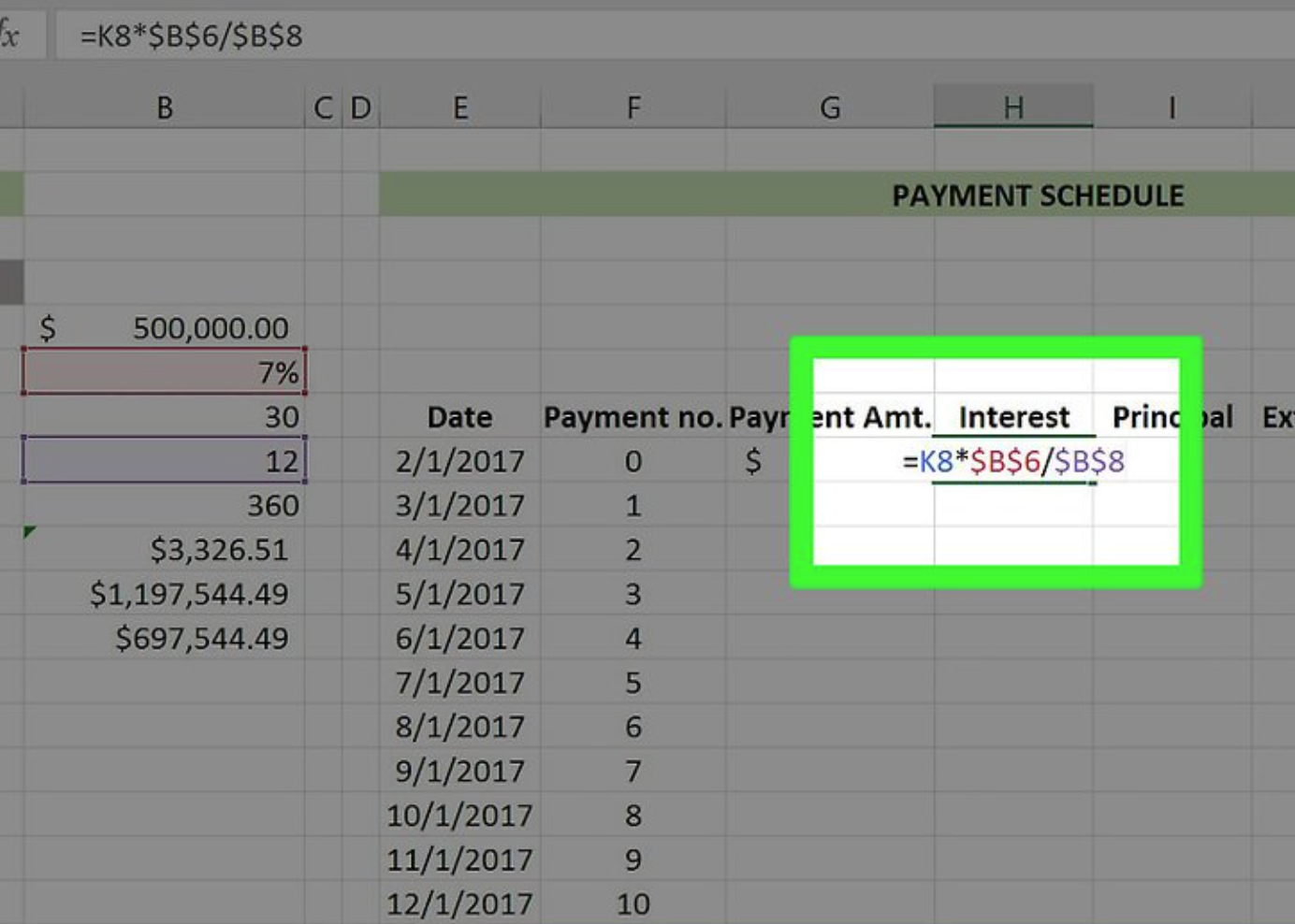Enter the formula for calculating your Interest value.
The formula used to calculate your interest value is as follows: “Total Loan*Annual Interest Rate/Number of Payments per Year”.
• This formula must be prefaced with a “=” sign in order to work.
• In the screenshots provided, the formula would look like this: “=K8*\$B\$6/\$B\$8” (without quotation marks).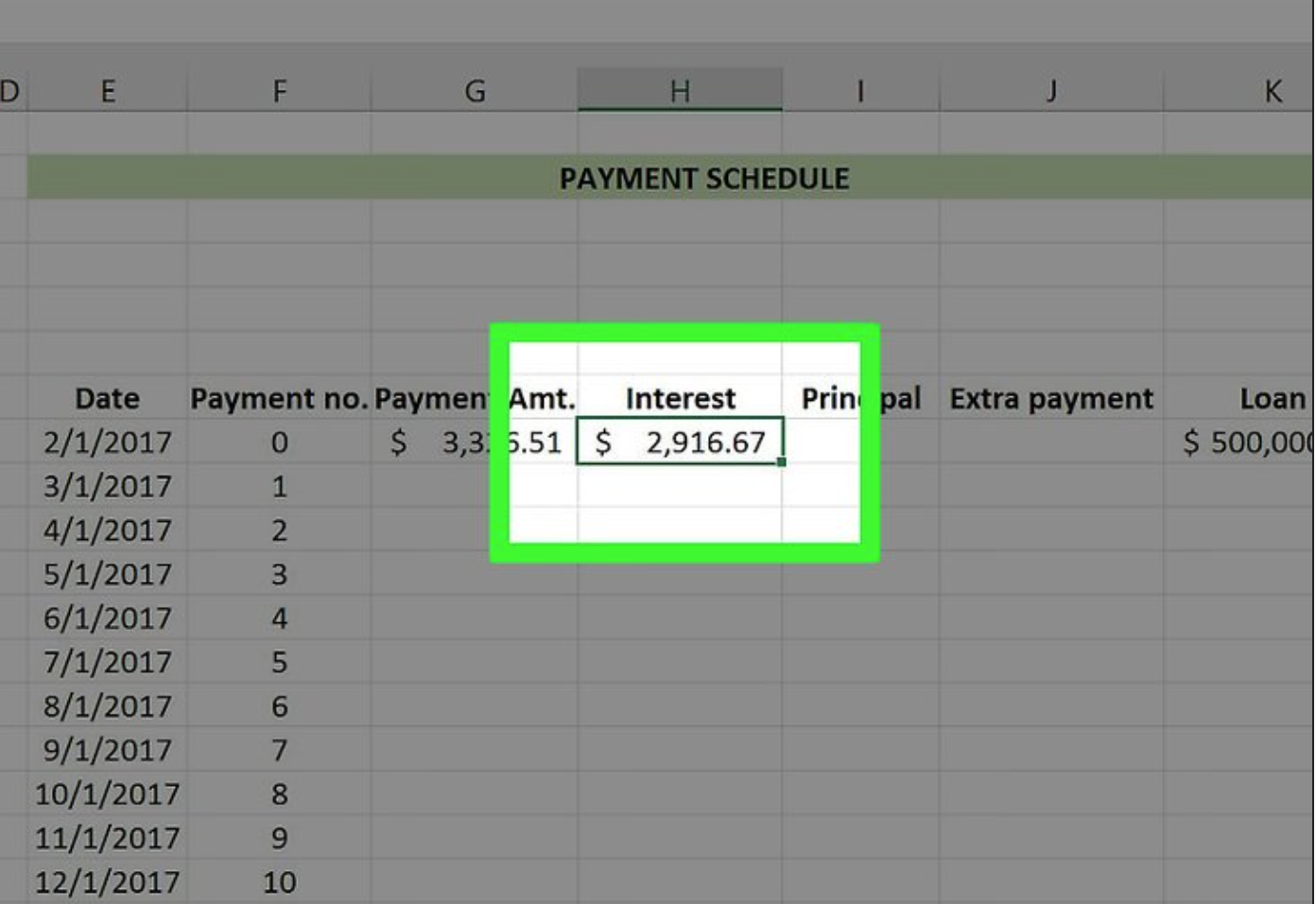Press Enter.

This will apply the Interest formula to the cell that has been selected.

To ensure that this formula is applied to all consecutive cells in this column, you must employ the “Fill” option that you previously used.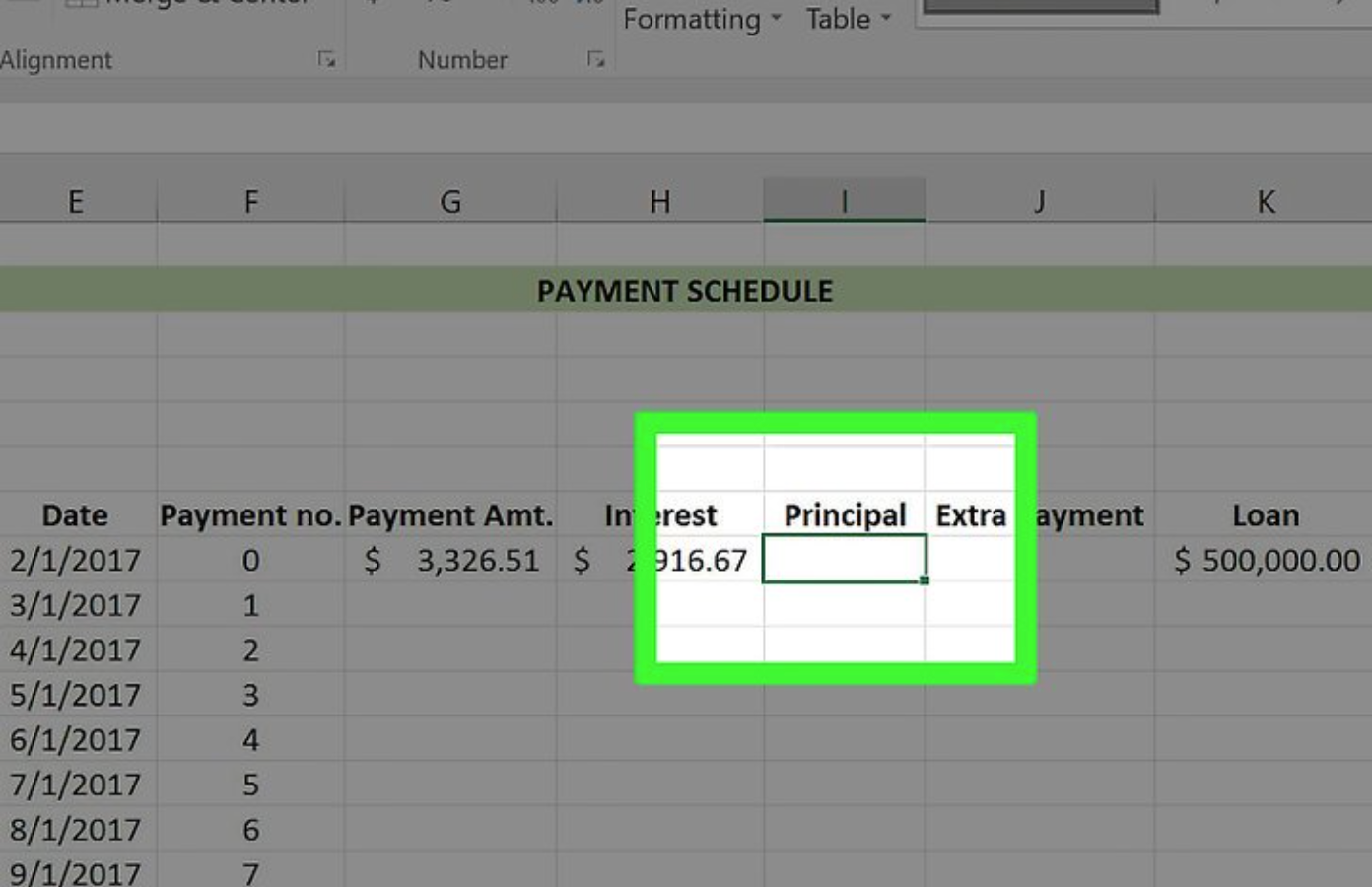Select the first empty cell in the “Principal” column.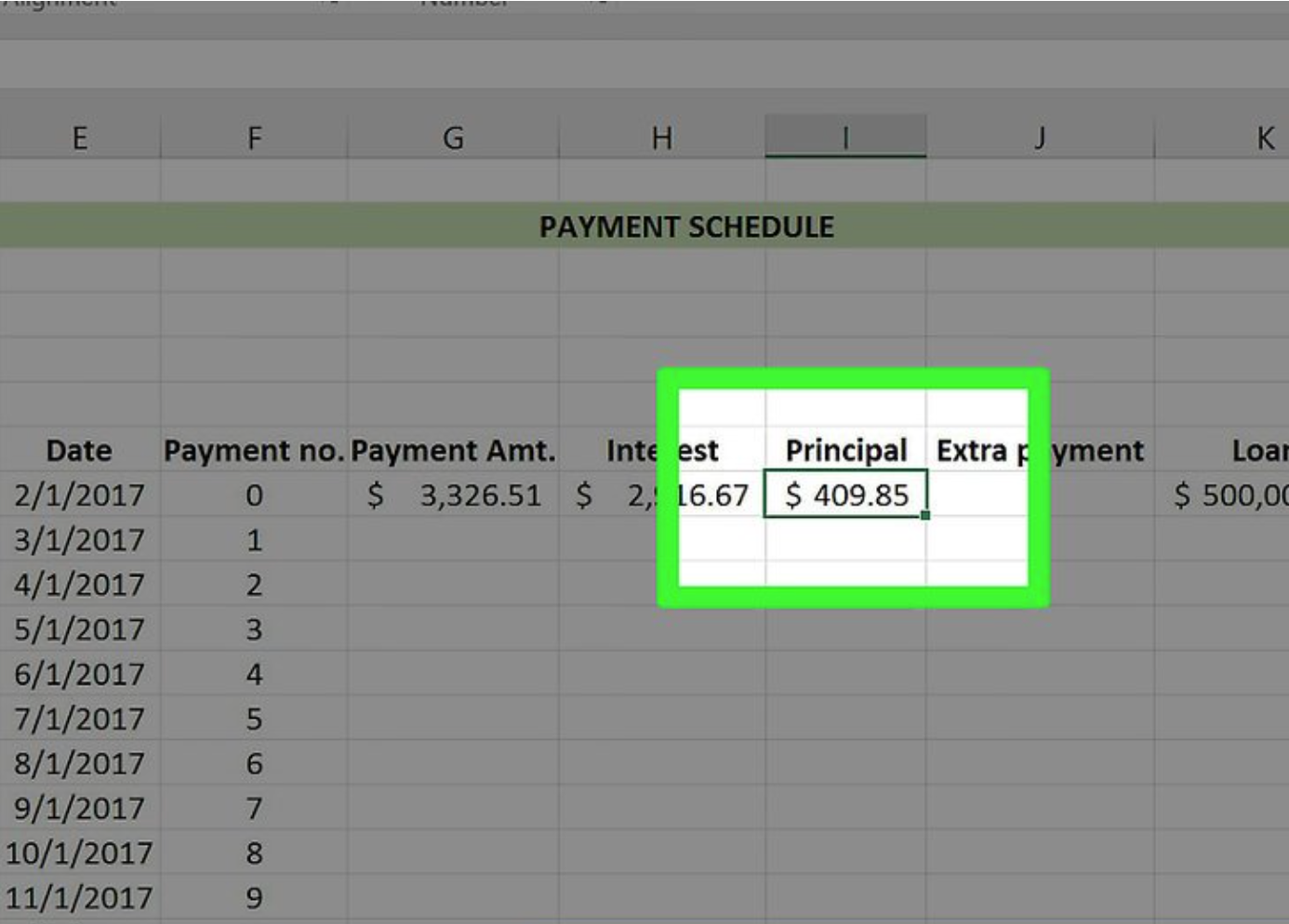Press Enter.

This will apply the Principal formula to the cell that has been selected.

To ensure that this formula is applied to all consecutive cells in this column, you must employ the “Fill” option that you previously used.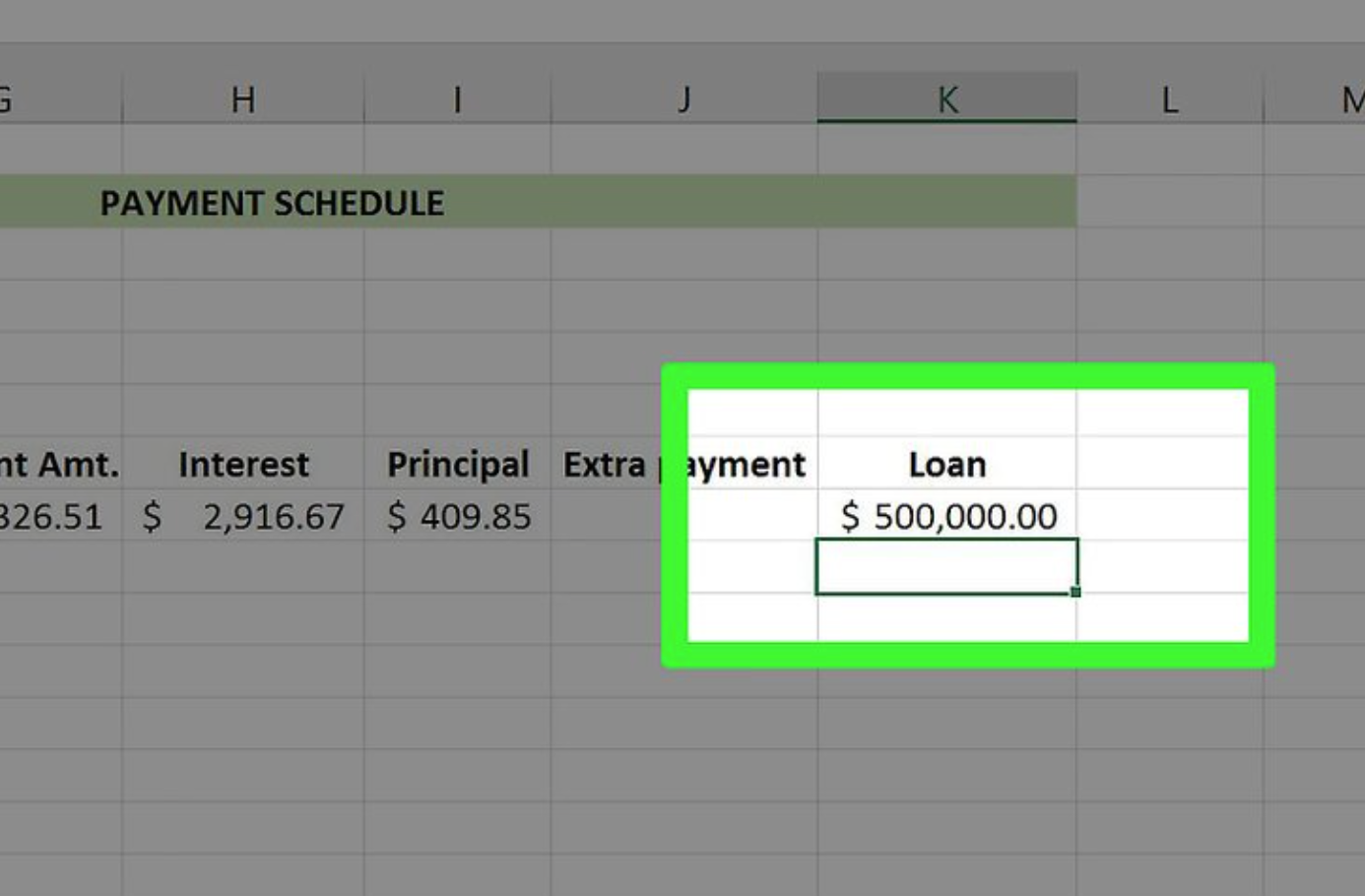It is necessary to select the first empty cell in the “Loan” column from the list. This sum should be equal to or less than the amount of the initial loan you acquired (e.g., the second cell in this column).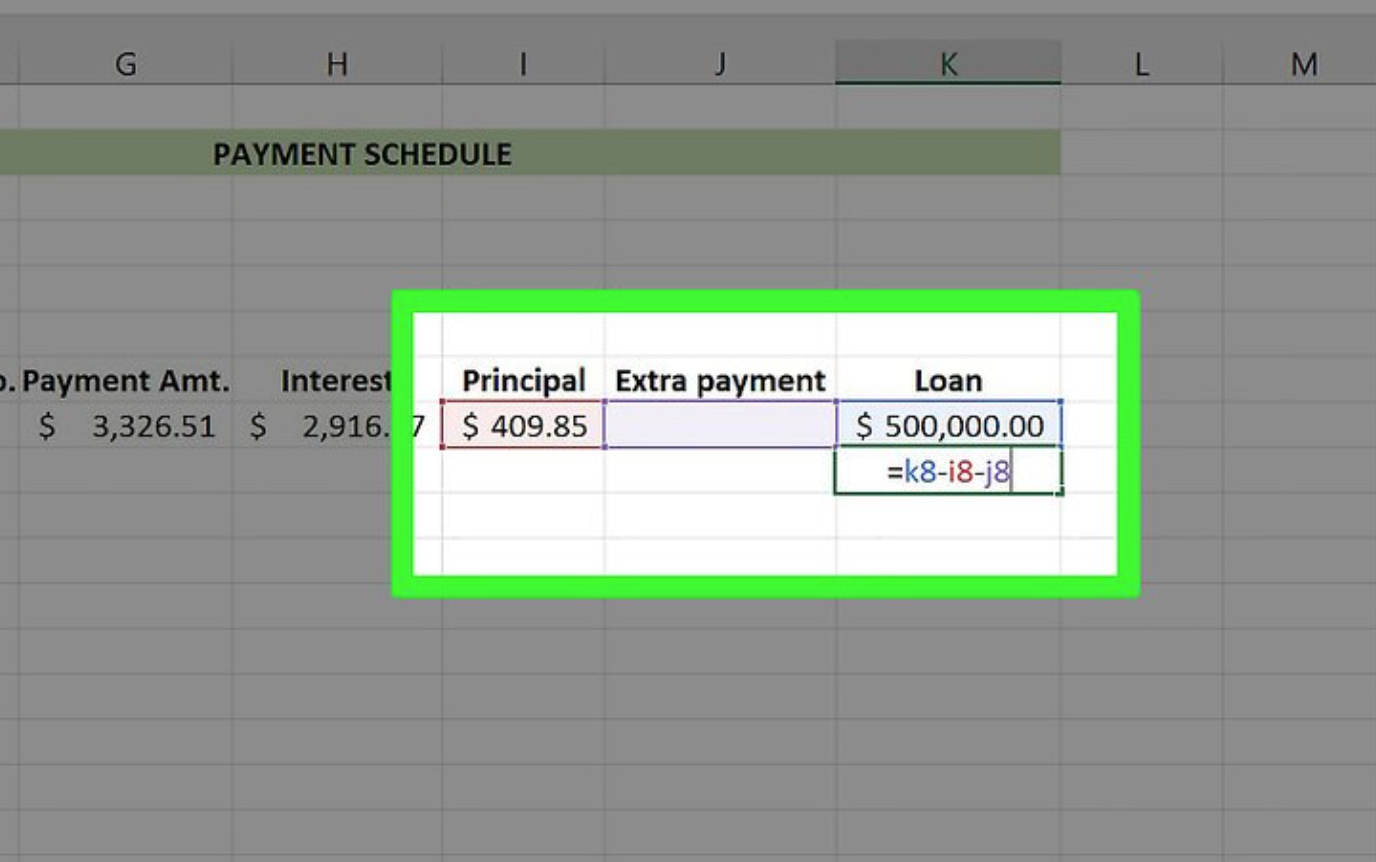#### Incorporate the Loan formula.

The following formula is used to determine the loan’s worth: “Loan“-“Principal“-“Extra.”

For the screenshots that were provided, you’d type “=K8-I8-J8” without quotation marks.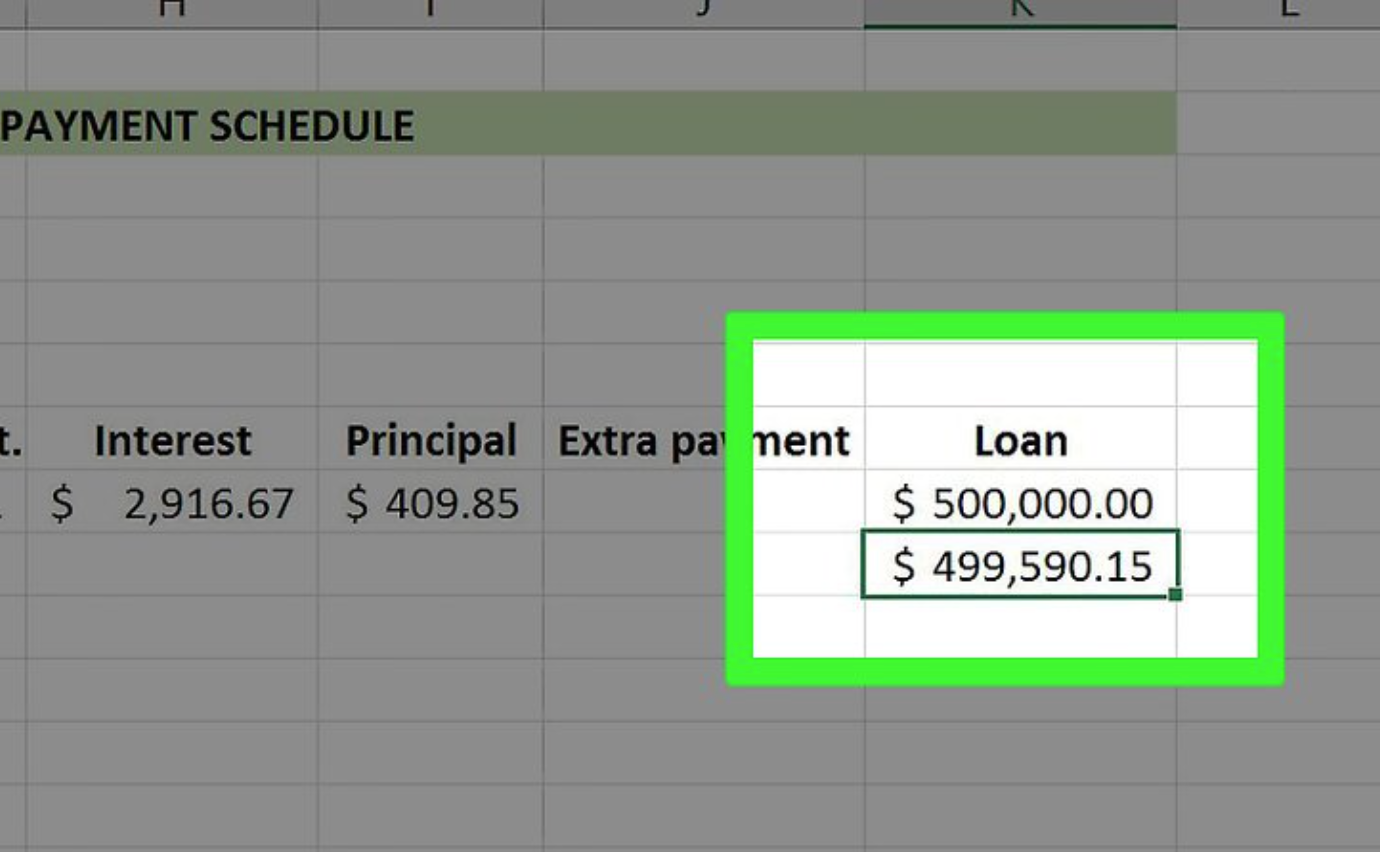Press Enter.

This will apply the Loan formula to the cell that you have selected.

Use the “Fill” tool, which you previously used to apply this formula to all subsequent cells in this column, in order to apply it to all subsequent cells in this column.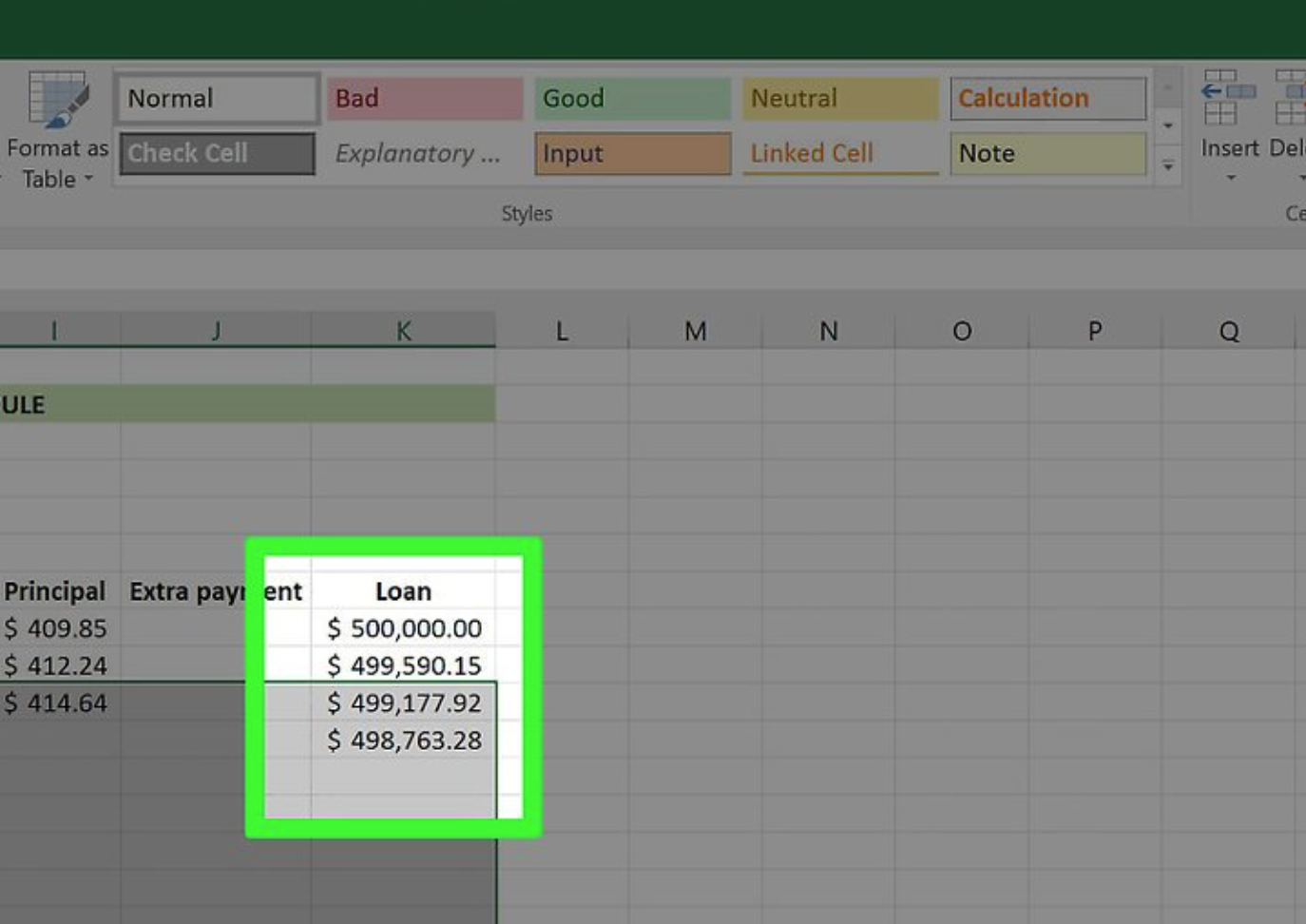Fill functions are used to fill in the blanks in your formula columns.

Your payment should be the same from the beginning to the end. The interest rate and loan amount should go down, but the values of the principal should go up in value.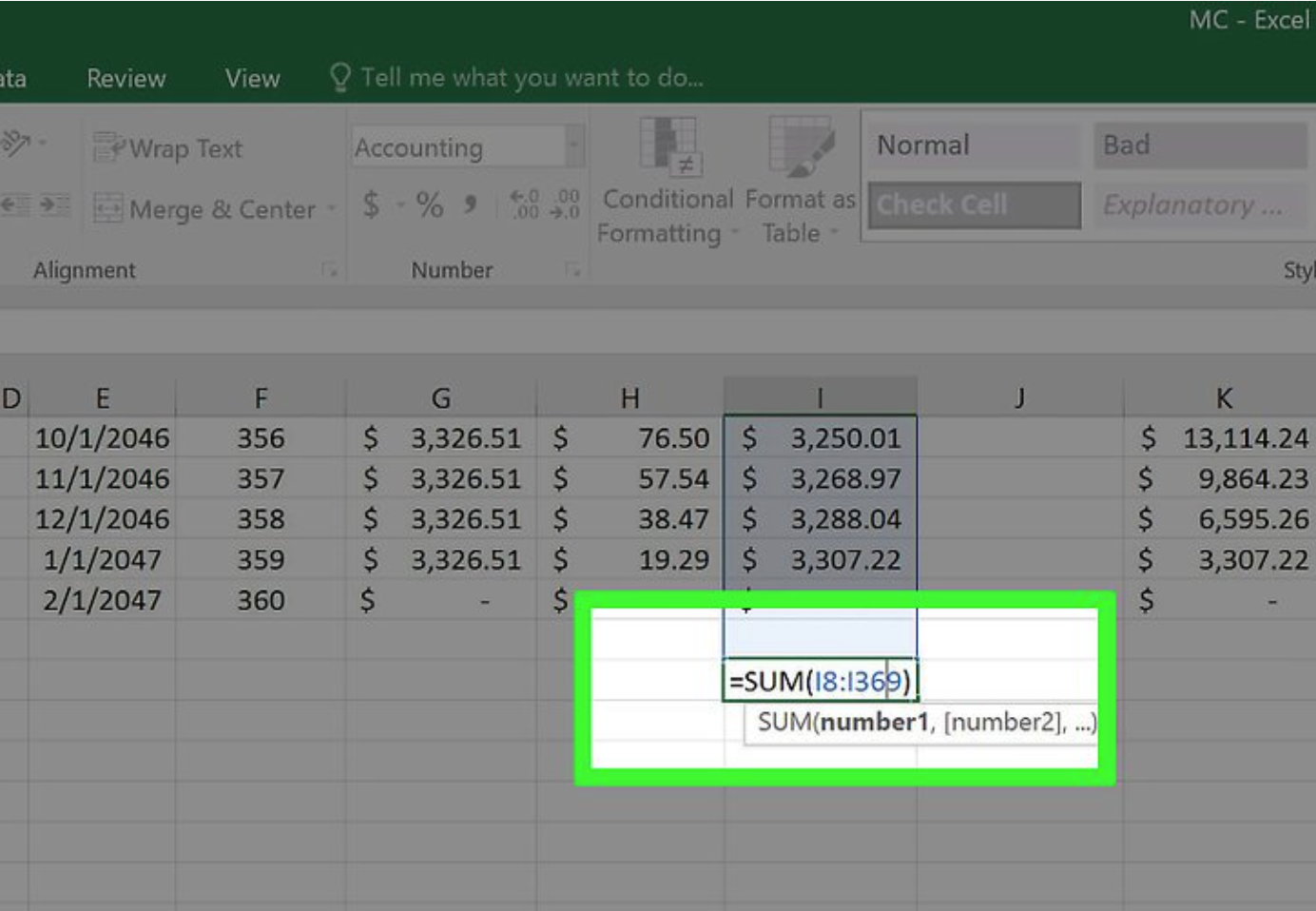The payment schedule should be added up. The total of the payments, interest, and principal may be found at the bottom of the table.

Compare these figures to the ones provided by your mortgage calculator. If they are the same, you have completed the formulas correctly.

• The amount of your principal should be the same as the amount of the original loan.
• The overall cost of the loan, as calculated by the mortgage calculator, should be the same as your monthly installments.
• Your interest rate should be the same as the interest rate calculated by the mortgage calculator.